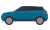A B C F G H J L N Q S T X Y Z
A

B

C

F

G

H

J

L

N

Q

S

T

X

Y

ZSUV 小型SUV 中型SUV 中大型SUV 大型SUV 豪华型SUV

A B C D F G H J K L M N O P Q R S T W X Y Z
A

AC Schnitzer
ALPINA
ARCFOX
B

BRABUS巴博斯

C

D

Dacia

DS
F

Fisker

G

GMC

H

J

Jeep

K

KTM

L

M

MINI

N

O

P

Q

R

S

SPIRRA
SSC

smart

SWM斯威汽车

T

W

WEY

X

Y

Z

[切换]
A B C F G H J L N Q S T X Y Z
A

B

C

F

G

H

J

L

N

Q

S

T

X

Y

Z51活动
51车展
•••••••【哈佛汽车】金秋钜惠团购会832人报名
•【一汽大众】大型团购会587人报名